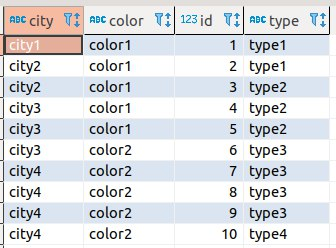# Create a percentage (%) metric of the total

I'm trying to create a metric that is a percentage of a given total.

Let's suppose the following table:I want to create the metric % of Type 3, that basically is (pseudo-code):

``````% Type 3 = CountRows(type == "type3") / CountRows(*)
``````

Without any filter, I would have:

``````% Type 3 = 4 / 10 = 0.4
``````

And filtering by `city4`, I would have:

``````% Type 3 (city == "city4") = 3 / 10 = 0.3
``````

How can I do that ?

Best Regards.

For your first example without filter, you can create a Custom Expression:
https://www.metabase.com/learn/building-analytics/notebook-editor/custom-expressions.html
`CountIf([Type] = "type3") / Count`

But for the example with filters, you would have to create that in SQL, since the Notebook editor does not have a way to do that currently.